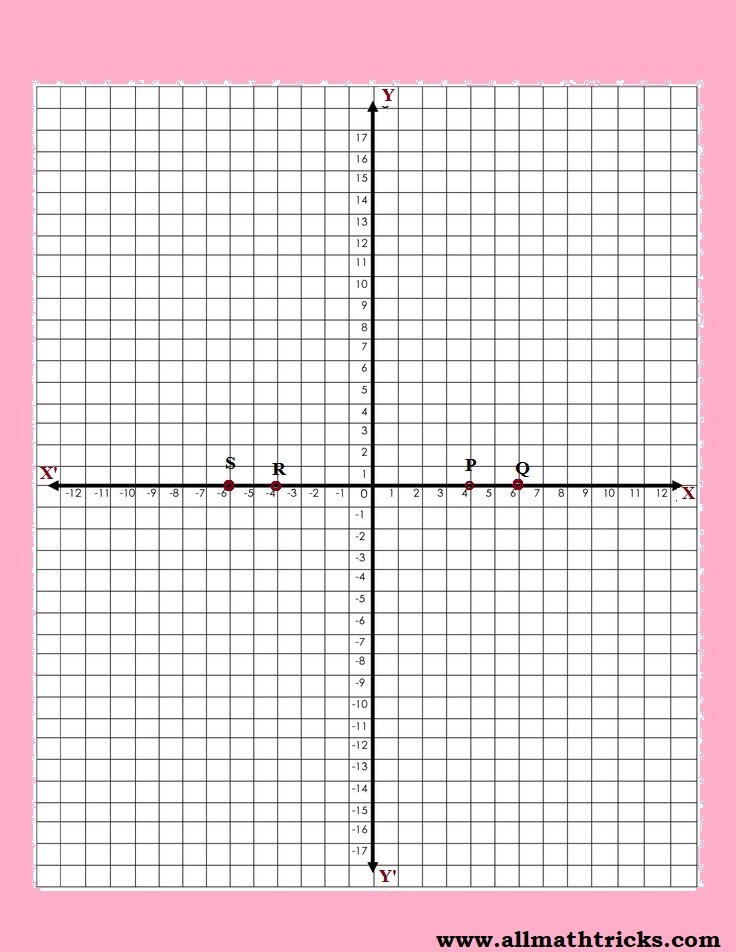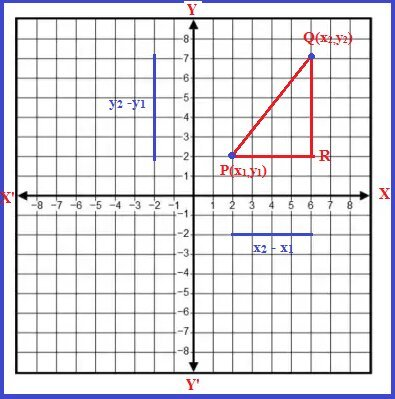# Distance formula geometry | Distance between two points on Coordinate plane

This article provided formulas with examples for distance formula in coordinate geometry. i.e. Distance between two points on coordinate plane formula with derivation also given different types of examples.

### What is the distance formula in coordinate geometry?

The distance formula is used to measure the distance (say d) between two points in the coordinate plane. If we considered the coordinates of the two points are A(x1, y1) and B(x2, y2) then the distance between two points ‘d’ is equal to the length of the straight line connected between these two coordinates in the plane.

#### a)The distance between points which are lying on X-axis or Y-axis

In this case, we can calculate distance easily. It is equal to the difference between the x-coordinates.

Let us consider two points P(x1, 0), Q(x2, 0)

These two points lie on X-axis because the values of y-coordinates are zero in both points.

Distance between P and Q is |x2 − x1| units

Let us consider two points A(0, y1), B(0, y2)

These two points lie on Y-axis because the values of the X-coordinates are zero in both points.

Distance between A and B is |y2 − y1| units

Note: Generally, we never say the distance in negative values.Example-1:

What is the distance between P(4, 0) and Q(6, 0)?

These two points are lying on X-axis due to the values of y-coordinates are zero.

The distance between P and Q is the difference in the value of x-coordinates.

6 -4 = 2 units

Example-2:

What is the distance between R(-4, 0) and S(-6, 0)?

The distance between R and S is the difference in the value of x-coordinates.

-6 -(-4) =| – 2| = 2 units

Example-3:

What is the distance between A(0, 2) and B(0, 9)?

These two points lie on Y-axis because the values of y-coordinates are zero.

The distance between A and B is the difference in the value of y-coordinates.

9 – 2 =| 7| = 7 units

Example-4:

What is the distance between C(0, -9) and D(0, -5)?

The distance between C and B is the difference in the value of y-coordinates.

– 9 – (-5) =| -4| = 4 units

Distance between two points on a line parallel to the coordinate axis

#### b)The distance between points which are parallel to X-axis or Y-axis

Let Considered two points of P(x1, y1) and Q(x2, y1).

Here the y-coordinates are equal in both points. Hence these points are lies on a line and also this line is parallel to X-axis.

Distance between two points  P & Q is equal to the difference between x-coordinates.
i.e PQ = |x2 − x1|

Note: Generally, we never say the distance in negative values.

Let Considered two points of R(x1, y1) and S(x1, y2).

Here the x-coordinates are equal in both points. Hence these points are lies on a line and also this line is parallel to Y-axis.

Distance between two points  R & S is equal to the difference between y-coordinates.
i.e RS = |y2 − y1|Example-5:

P and Q are two points given by (6, 4), and (−2, 4) respectively. Find the distance between P & Q.

Here both y-coordinates are the same. Hence the distance between P and Q is the difference in the value of x-coordinates.

i.e -2 – 6 =| -8| = 8 units

Example-6:

R and S are two points given by (3, -4), and (3, 4) respectively. Find the distance between R & S.

Here both x-coordinates are the same. Hence the distance between R and S is the difference in the value of y-coordinates.

i.e 4 -(-4) =| 8 | = 8 units

#### c) Distance between two points on a line in the X-Y plane ( Distance Formula)

Let P(x1, y1) and Q(x2, y2) be any two points in a plane.

As shown in the below figure.

By using P & Q points we can from ΔPRQ (right angle triangle)

According to Pythagoras’ theorem

PQ2 = PR2 + QR2

PR = x2-x1

QR = y2 – y1

PQ2 = ( x2-x1)2 + (y2 – y1)2

Hence, the distance between the points P & Q isThis formula is also called a distance formulaExample -7

Find the distance between two points P(2, 6) and Q(6, 9)

Here we compare these points with P(x1, y1) and Q(x2, y2)
x1 = 2,  x2 = 6,

y1 = 6, y2 = 9

Now using the distance formulaPQ = √25 = 5 units

#### d) Collinear points in the coordinate plane

Let P(x1, y1) , Q(x2, y2) & R(x3, y3) are be any three points in a plane.

The distances of their points are PQ, QR & RP

If the sum of any two distances is equal to another distance then their points lie on a straight line. Hence these points are called collinear points

i.e PQ + QR = RP (or) QR + RP = PQ (or) PQ + RP = QR

Example -8

Verify that the points P(1, 5), Q(2, 3) and R(−2, −1) are collinear or not.

Here find the distance between the points PQ, QR & RP

PQ = √ (1)2 + (-2)2 = √5

QR = √ (-4)2 + (-4)2 = √(16 x 2) = 4 √2

RP = √ (-3)2 + (-6)2 = √45 = 3 √5

Here the sum of any two distances is not equal to another distance. So these points do not lie in a straight line.

Hence these points are not collinear points.

Example -9

Verify that the points P(2,7), Q(5,6) and R(8,5) are collinear or not.

Here find the distance between the points PQ, QR & RP

PQ = √ (3)2 + (-1)2 = √10

QR = √ (3)2 + (-1)2 = √10

RP = √ (6)2 + (-2)2 = √40 = 2 √10

Here PQ + QR = RP

The sum of PQ & QR is equal to RP. So points P, Q & R lies in a straight line. Hence these points are called collinear points.

Example – 10

Are the points A(2,5), B(3,8) & C(4,5) form a triangle?

Here find the distance between the points AB, BC & CA

AB = √ (1)2 + (3)2 = √10

BC = √ (1)2 + (-3)2 = √10

CA = √ (2)2 + (0)2 = √ 4

The sum of any two of these distances is greater than the third.

Hence points A, B & C  form a triangle. Also, two sides of a triangle are equal. These three points form an isosceles triangle.

Example -11

Are the points P(5, −2), Q(6, 4) and R(7, −2) form an isosceles triangle?

Here find the distance between the points PQ, QR & RP

PQ = √ (1)2 + (6)2 = √37

BC = √ (1)2 + (-6)2 = √37

CA = √ (2)2 + (0)2 = √ 4

The points P, Q & R  form a triangle. Also, two sides of a triangle are equal. These three points form an isosceles triangle.

Example -12

Are the points P(b, 0), Q(−b, 0), R(0, b √3 ) form a equilateral triangle.

Here find the distance between the points PQ, QR & RP

PQ = √ (-2b)2 + (0)2 = 2b

QR = √ (b)2 + (b√3)2 = √4b2 = 2b

RP = √ (b)2 + (-b√3)2 = √4b2 = 2b

The points P, Q & R  form a triangle. Also, the three sides of the triangle are equal. These three points form an equilateral triangle.

#### Distance formula geometry practice problems:

1) What is the distance between P(2, 3) and Q(4, 5)?

A. 1    B. 2     C. 3     D. 4

2) Find the distance between two points C(-1, -2) and D(3, -4).

A. 3    B. 4     C. 5    D. 6

3) Verify that the points E(1, -2), F(2, -5) and G(-1, -8) are collinear or not.

A. Collinear     B. Not collinear

4) Are the points H(-5, -6), I(-7, -8) and J(-9, -10) form an isosceles triangle?

A. Yes       B. No

5) What is the distance between K(0, 0) and L(0, 5)?

A. 1       B. 2      C. 3      D. 4

6) Find the distance between two points M(-2, -3) and N(1, -6).

A. 3     B. 4       C. 5       D. 6

7) Verify that the points O(0, 0), P(1, √3) and Q(2√3, √3 + 1) are collinear or not.

A. Collinear     B. Not collinear

8) Are the points R(-1, -2), S(0, -4) and T(1, -6) form an isosceles triangle?

A. Yes       B. No

9) What is the distance between U(-4, -3) and V(-6, -7)?

A. √20    B. √24     C. √28     D. √32

10) Find the distance between two points W(0, -2) and X(-4, -6).

A. √20     B. √24     C. √28    D. √32

11) Verify that the points Y(-2,-1), Z(0,-2), and A(2,-3) are collinear or not.

A.Collinear        B.Not collinear

12) Are the points B(-5,-6), C(-7,-8), and D(-9,-10) form an isosceles triangle?

A.Yes         B.No

Here are the correct answers to the above questions:

1. B
2. C
3. Not collinear
4. No
5. B
6. A
7. Not collinear
8. Yes
9. √20
10. √20
11. Collinear
12. No